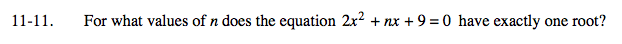### Home > A2C > Chapter Ch11 > Lesson 11.1.1 > Problem11-11

11-11.

For what values of n does the equation 2x2 + nx + 9 = 0 have exactly one root? Homework Help ✎Make the discriminant equal to zero.

n2 − 4(2)(9) = 0